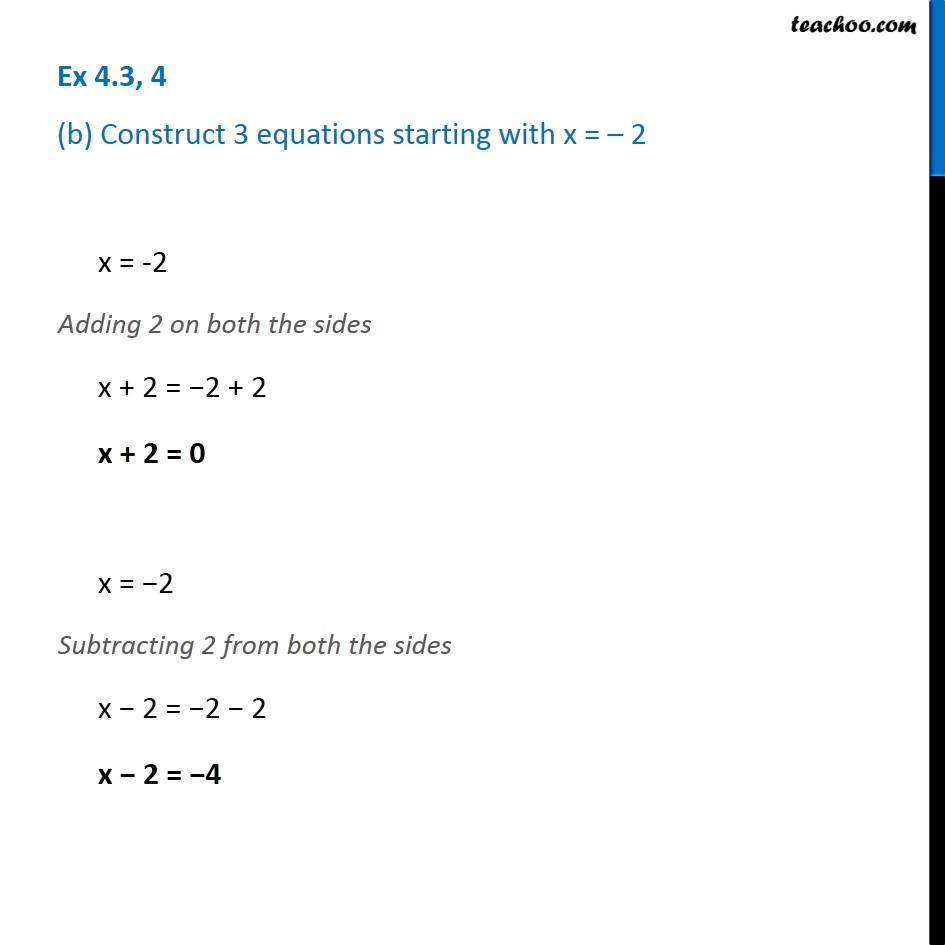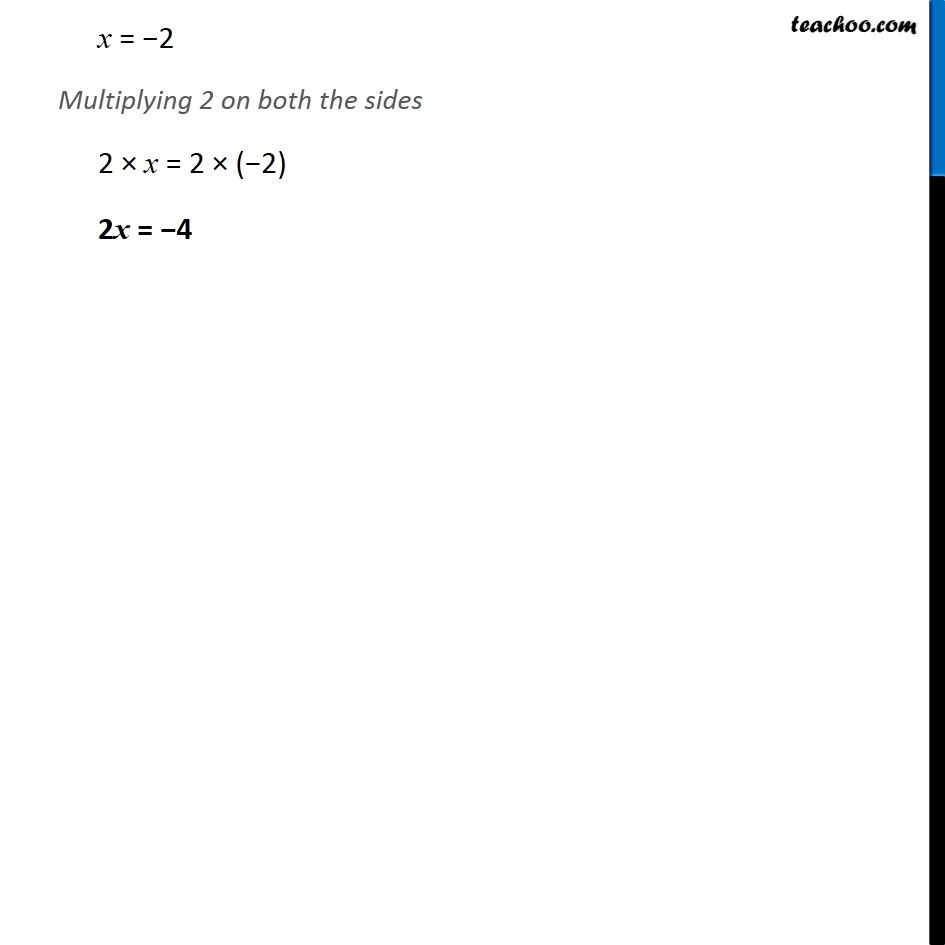Ex 4.3

Chapter 4 Class 7 Simple Equations
Serial order wiseGet live Maths 1-on-1 Classs - Class 6 to 12

### Transcript

Ex 4.3, 4 (b) Construct 3 equations starting with x = – 2x = -2 Adding 2 on both the sides x + 2 = −2 + 2 x + 2 = 0 x = −2 Subtracting 2 from both the sides x − 2 = −2 − 2 x − 2 = −4 x = −2 Multiplying 2 on both the sides 2 × x = 2 × (−2) 2x = −4